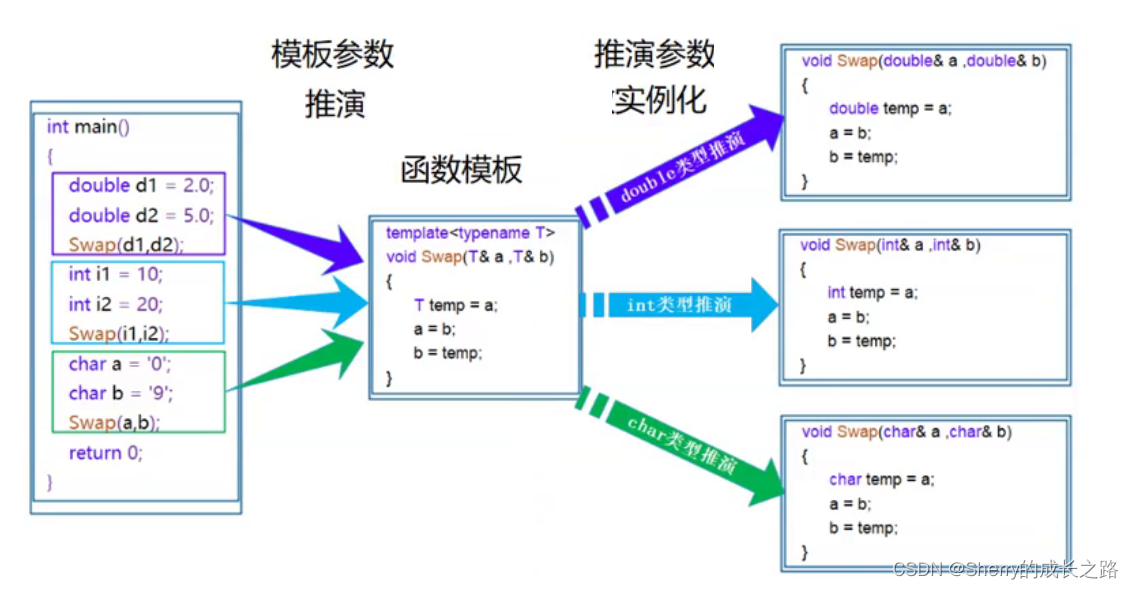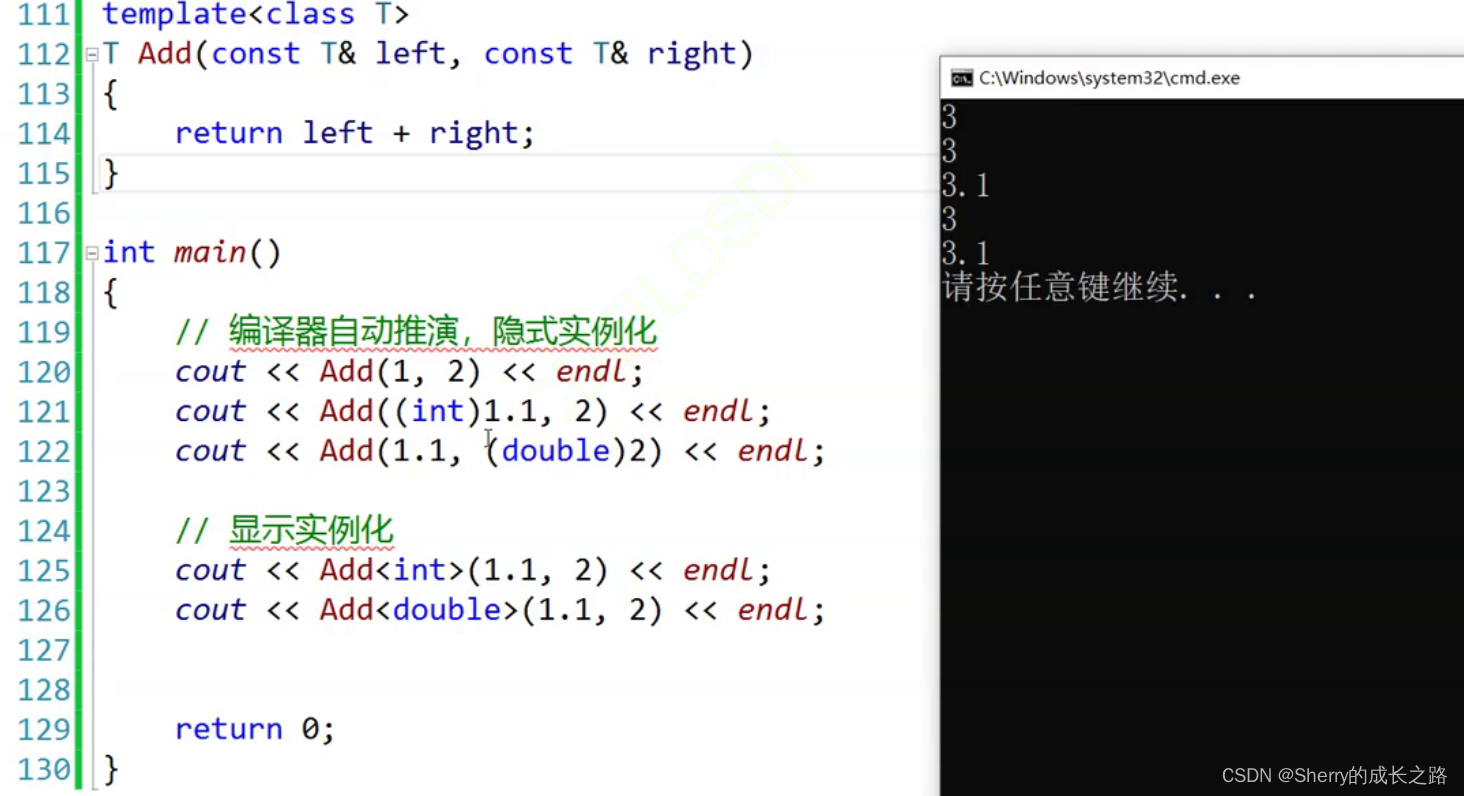## 【C++初阶】C++——模板初阶与泛型编程

article2023/9/27 9:50:47/​📝个人主页：@Sherry的成长之路
🏠学习社区：Sherry的成长之路（个人社区）
📖专栏链接：C++初阶
🎯长路漫漫浩浩，万事皆有期待

• 1. 泛型编程
• 2. 函数模板
• 3. 函数模板的原理
• 4. 函数模板的实例化
• 4.1 隐式实例化
• 4.2 显示实例化
• 5. 函数模板的匹配原则
• 6. 类模板
• 7. 类模板的实例化
• 8.总结：

# 1. 泛型编程

``````// 交换两个整型
void Swapi(int* p1, int* p2)
{
int tmp = *p1;
*p1 = *p2;
*p2 = tmp;
}
// 交换两个双精度浮点型
void Swapd(double* p1, double* p2)
{
double tmp = *p1;
*p1 = *p2;
*p2 = tmp;
}
``````

``````// 交换两个整型
void Swap(int& x, int& y)
{
int tmp = x;
x = y;
y = tmp;
}
// 交换两个双精度浮点型
void Swap(double& x, double& y)
{
double tmp = x;
x = y;
y = tmp;
}
``````

C++的函数重载使得用于交换不同类型变量的函数可以拥有相同的函数名，并且传参使用引用传参，使得代码看起来更加简单。

1、重载的多个函数仅仅只是类型不同，代码的复用率比较低，只要出现新的类型需要交换，就需要新增对应的重载函数。
2、代码的可维护性比较低，其中一个重载函数出现错误可能意味着所有的重载函数都出现了错误。

如果在C++中，也能够存在这样一个模具，通过给这个模具填充不同颜色的材料(类型)，从而得到形状相同但颜色不同的月饼(生成具体类型的代码)，那将会大大减少代码的冗余。

# 2. 函数模板

函数模板代表了一个函数家族，该函数模板与类型无关，在使用时被参数化，根据实参类型产生函数的特定类型版本。

template<typename T1,typename T2,…,typename Tn>

{
//函数体
}

``````template<typename T>
void Swap(T& x, T& y)
{
T tmp = x;
x = y;
y = tmp;
}
``````

`注意`：typename是用来定义模板参数的关键字，也可以用class代替，但是不能用struct代替。

# 3. 函数模板的原理# 4. 函数模板的实例化

## 4.1 隐式实例化

``````#include <iostream>
using namespace std;
template<typename T>
T Add(const T& x, const T& y)
{
return x + y;
}
int main()
{
int a = 10, b = 20;
int c = Add(a, b); //编译器根据实参a和b推演出模板参数为int类型
return 0;
}
``````

`注意`：使用模板时，编译器一般不会进行类型转换操作。所以，以下代码将不能通过编译：

``````	int a = 10;
double b = 1.1;
``````

此时，我们有两种处理方式，第一种就是我们在传参时将b强制转换为int类型，第二种就是使用下面说到的显示实例化。## 4.2 显示实例化

``````#include <iostream>
using namespace std;
template<typename T>
T Add(const T& x, const T& y)
{
return x + y;
}
int main()
{
int a = 10;
double b = 1.1;
int c = Add<int>(a, b); //指定模板参数的实际类型为int
return 0;
}
``````

`注意`：使用显示实例化时，如果传入的参数类型与模板参数类型不匹配，编译器会尝试进行隐式类型转换，如果无法转换成功，则编译器将会报错。

# 5. 函数模板的匹配原则

``````#include <iostream>
using namespace std;
//专门用于int类型加法的非模板函数
int Add(const int& x, const int& y)
{
return x + y;
}
//通用类型加法的函数模板
template<typename T>
T Add(const T& x, const T& y)
{
return x + y;
}
int main()
{
int a = 10, b = 20;
int c = Add(a, b); //调用非模板函数，编译器不需要实例化
return 0;
}
``````

``````#include <iostream>
using namespace std;
//专门用于int类型加法的非模板函数
int Add(const int& x, const int& y)
{
return x + y;
}
//通用类型加法的函数模板
template<typename T1, typename T2>
T1 Add(const T1& x, const T2& y)
{
return x + y;
}
int main()
{
int a = Add(10, 20); //与非模板函数完全匹配，不需要函数模板实例化
return 0;
}
``````

``````#include <iostream>
using namespace std;
template<typename T>
T Add(const T& x, const T& y)
{
return x + y;
}
int main()
{
int a = Add(2, 2.2); //模板函数不允许自动类型转换，不能通过编译
return 0;
}
``````

# 6. 类模板

template<class T1,class T2,…,class Tn>
class 类模板名
{
//类内成员声明
};

``````template<typename T>
class Stack
{
public:
Stack(size_t capacity = 4)
:_a(nullptr)
,_capacity(0)
,_top(0)
{
if (capacity > 0)
{
_a = new T[capacity];
_capacity = capacity;
_top = 0;
}
}
~Stack()
{
delete[] _a;
_a = nullptr;
_capacity = _top = 0;
}
void Push(const T& x)
{
if (_top==_capacity)
{
size_t newcapacity = _capacity == 0 ? 4 : _capacity * 2;
T* tmp = new T[newcapacity];
if (_a)
{
memcpy(tmp, _a, sizeof(T)*(_top));
delete[] _a;
}

_a = tmp;
_capacity = newcapacity;
}
_a[_top] = x;
++_top;
}
void Pop()
{
assert(_top > 0);
--top;
}
bool Empty()
{
return _top == 0;
}
T& Top()
{
assert(_top > 0);

return _a[_top - 1];
}
private:
T* _a;
size_t _top;
size_t _capacity;
};
``````

`注意`：类模板中的成员函数若是放在类外定义时，需要加模板参数列表。

``````template<typename T>
class Stack
{
public:
Stack(size_t capacity = 4)
:_a(nullptr)
, _capacity(0)
, _top(0)
{
if (capacity > 0)
{
_a = new T[capacity];
_capacity = capacity;
_top = 0;
}
}
~Stack()
{
delete[] _a;
_a = nullptr;
_capacity = _top = 0;
}
void Pop()
{
assert(_top > 0);
--top;
}
bool Empty()
{
return _top == 0;
}
T& Top()
{
assert(_top > 0);
return _a[_top - 1];
}
private:
T* _a;
size_t _top;
size_t _capacity;
};
//类模板中的成员函数在类外定义，需要加模板参数列表
template<typename T>
void Stack<T>::Push(const T& x)
{
if (_top == _capacity)
{
size_t newcapacity = _capacity == 0 ? 4 : _capacity * 2;
T* tmp = new T[newcapacity];
if (_a)
{
memcpy(tmp, _a, sizeof(T) * (_top));
delete[] _a;
}

_a = tmp;
_capacity = newcapacity;
}
_a[_top] = x;
++_top;
}
``````

# 7. 类模板的实例化

``````    //Stack不是真正的类，Stack<int>和Stack<double>才是真正的类
Stack<int> st1;
Stack<double> st2;
``````

`注意`：类模板名字不是真正的类，而实例化的结果才是真正的类。

# 8.总结：### 自学网络安全最细规划（建议收藏）

01 什么是网络安全 网络安全可以基于攻击和防御视角来分类&#xff0c;我们经常听到的 “红队”、“渗透测试” 等就是研究攻击技术&#xff0c;而“蓝队”、“安全运营”、“安全运维”则研究防御技术。 无论网络、Web、移动、桌面、云等哪个领域&#xff0c;都有攻与防两面…

### 8项seo的日常工作

SEO的日常工作涵盖了一系列任务和活动&#xff0c;旨在优化网站以提高在搜索引擎中的排名和可见性。 以下是SEO的日常工作内容&#xff1a; 关键词研究和优化&#xff1a;定期进行关键词研究&#xff0c;寻找与目标受众和业务相关的热门关键词。优化网站内容、标题、元描述和链…

### 这些脑洞大开的论文标题，也太有创意了O(∩_∩)O

microRNAs啊microRNAs&#xff0c;谁是世界上最致命的髓母细胞瘤microRNAs&#xff1f; 这个标题很容易让人联想到白雪公主后妈说的那句话&#xff1a;Mirror mirror on the wall, who is the fairest of them all? 02 一氧化碳&#xff1a;勇踏NO未至之境 NO 指 nitric oxide…

### 合并两个有序链表（java）

leetcode 21题&#xff1a;合并两个有序链表 题目描述解题思路&#xff1a;链表的其它题型。 题目描述 leetcode21题&#xff1a;合并两个有序链表 将两个升序链表合并为一个新的 升序 链表并返回。新链表是通过拼接给定的两个链表的所有节点组成的。 示例&#xff1a; 输入&…

### Word控件Spire.Doc 【其他】教程(4)：在 Word 中插入上标和下标

Spire.Doc for .NET是一款专门对 Word 文档进行操作的 .NET 类库。在于帮助开发人员无需安装 Microsoft Word情况下&#xff0c;轻松快捷高效地创建、编辑、转换和打印 Microsoft Word 文档。拥有近10年专业开发经验Spire系列办公文档开发工具&#xff0c;专注于创建、编辑、转…